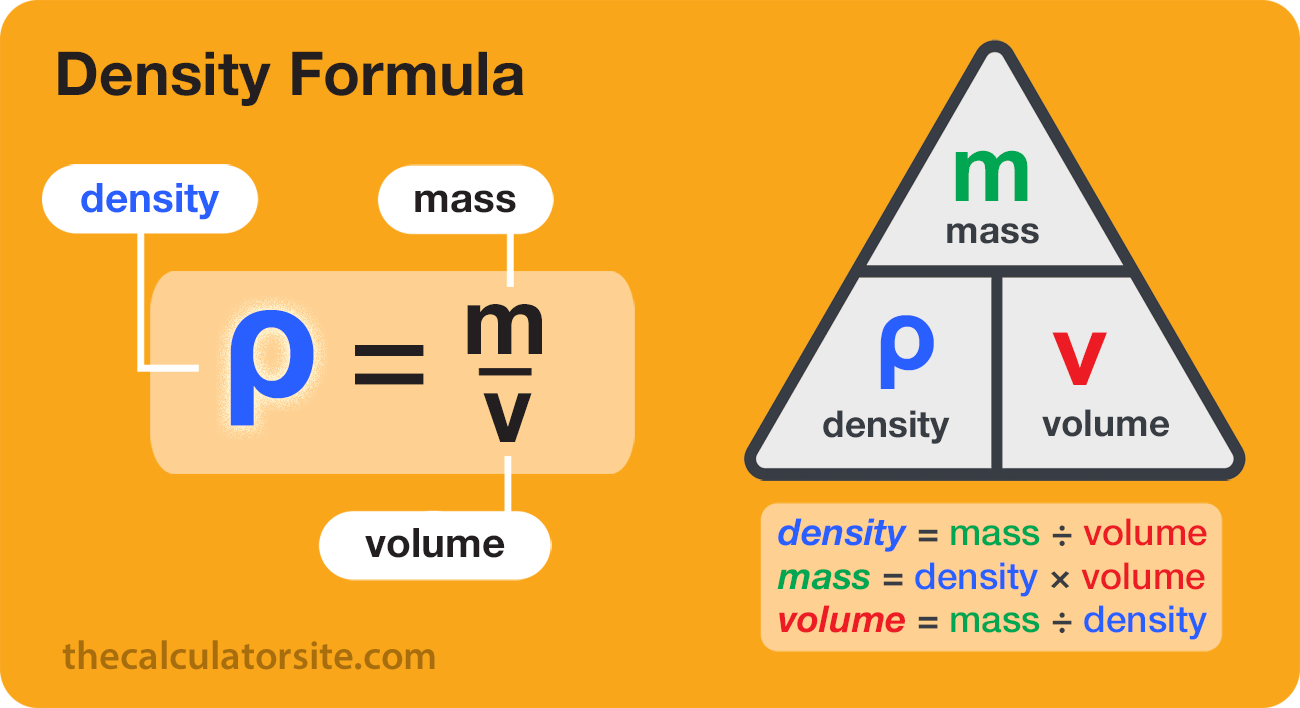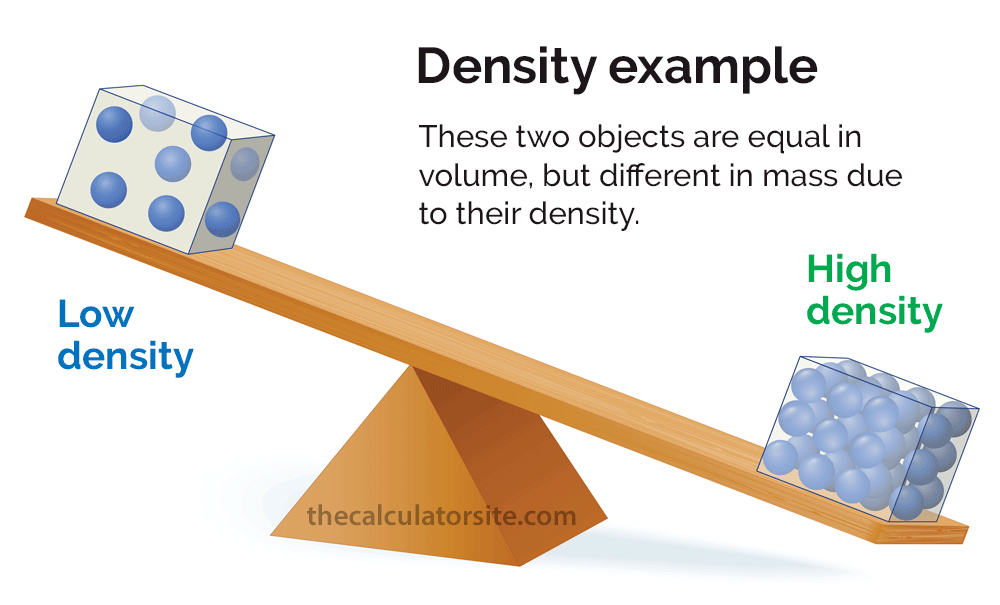# Density Formula - How To Calculate Density

| Last update: 20 September 2023

The formula for calculating density is ρ = m/V, where p is the density, m is the mass and V is the volume. Density (p) is equal to mass (m) divided by volume (V).As there are three elements to the formula, it can be expressed in other ways depending on which element you want to calculate.

To calculate density: ρ = m/V To calculate mass: m = ρV To calculate volume: V = m/ρ

If you've ever been fooled by the old question, 'Which weighs more: a pound of feathers or a pound of lead?' then you wouldn't be alone. Although the feathers and the lead both weigh exactly the same (um, a pound), their density is wildly different, and we can sometimes mentally conflate weight and density - even though they're different concepts. So - hey - don't feel dense. Read on to find out exactly what density is, how it works, and how to calculate density.

## What is density?

Density is the mass per volume - not just the straight-up mass. So if the question was, 'Which weighs more: a 500ml jug full of feathers or a 500ml jug full of lead?' then the answer would be the lead. For an equal amount of space it takes up (volume), lead weighs much more than the feathers (mass).

Density can also be thought of as how compacted or compressed a substance is. A pound of feathers is filled with airy space, so it's not very dense at all, while a pound of lead feels much more solid, so it's more dense. This is why you should never comment on your Auntie Mabel's sponge cake by describing it as dense: she was probably going for something a little more airy.

Density is also what makes things float or sink. When you mix two or more substances, the most dense substance sinks to the bottom, whilst the least dense substance is more buoyant and floats to the top. Try mixing oil and water and see how they separate into layers, with the less dense oil on top.## How do you calculate density?

Always keep an eye on your units: it's standard to use g or kg per cm³ or m³, so use our unit converters if your measurements are in different units.

To calculate density, you divide the mass by the volume:

Density = Mass ÷ Volume

Density is often written in mathematics as the symbol p or D. Mass can be written as m, and volume can be written as V. So if you want to be fancy, the formula looks like this:

ρ = m/V

(Volume is to do with filling space: check out our guide to volume and weight and our volume calculator if you're not yet confident with it.)

So, if you have a kilogram of cheese with the volume of 0.0002m³, then not only are you in for some weird and wonderful dreams, but you also have cheese with a density of 5000kg/m³ (ρ = 1kg ÷ 0.0002m³).

Try our density calculator to make it all a piece of cake. Hopefully even better than Auntie Mabel's lemon sponge...

ρ=m/V
Calculation results will appear here.

For assistance with converting between units of mass and volume, give our weight and volume converter a try.

## Some examples

Why not fill up your bath and try out some different materials to see what floats or sinks? Just no electricals, please. ⚡

Water has a density of 1000kg/m³, therefore anything that floats in water has less density than that, and anything that sinks has a greater density (for example, cooking oil is around 920kg/m³; tin is 7310kg/m³; cork is 240kg/m³; and Aerogel, one of the least dense solids we know of, has a density of 1kg/m³). So how come people float so easily in the Dead Sea? Because it's not just water. It has an incredibly high amount of salt in it, creating an overall density of 1240kg/m³.